• +91 9971497814
• info@interviewmaterial.com

# RD Chapter 11- Co-ordinate Geometry Ex-MCQS Interview Questions Answers

### Related Subjects

Question 1 :
If all the three angles of a triangle are equal, then each one of them is equal to
(a) 90°
(b) 45°
(c) 60°
(d) 30°

Sum of three angles of a triangle = 180°
Eachangle =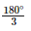= 60° (c)

Question 2 :
If two acute angles of a right triangle are equal, then each acute is equal to
(a) 30°
(b) 45°
(c) 60°
(d) 90°

In a right triangle, one angle = 90°
∴ Sum of other two acute angles = 180° – 90° = 90°
∵ Both angles are equal
∴ Each angle will be =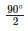= 45° (b)

Question 3 :
An exterior angle of a triangle is equal to 100° and two interior opposite angles are equal. Each of these angles is equal to
(a) 75°
(b) 80°
(c) 40°
(d) 50°

In atriangle, exterior angles is equal to the sum of its interior opposite angles
Sum ofinterior opposite angles = 100°
Bothangles are equal
Eachangle will be =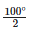= 50° (d)

Question 4 :
If one angle of a triangle is equal to the sum of the other two angles, then the triangle is
(a) an isosceles triangle
(b) an obtuse triangle
(c) an equilateral triangle
(d) a right triangle

Let ∠A, ∠B, ∠C be the angles of a ∆ABC and let ∠A = ∠B + ∠C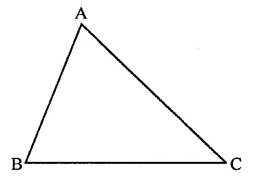But ∠A + ∠B + ∠C = 180°
( Sum of angles of a triangle)
∴ ∠A + ∠A = 180° ⇒ 2∠A = 180°
⇒ ∠A =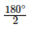= 90°
∴ ∆ is a right triangle (d)

Question 5 :
Side BC of a triangle ABC has been produced to a point D such that ∠ACD = 120°. If ∠B = 12∠A, then ∠A is equal to
(a) 80°
(b) 75°
(c) 60°
(d) 90°

Side BC of ∆ABC is produced to D, then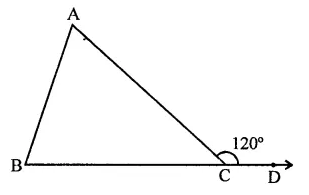Ext. ∠ACB = ∠A + ∠B
(Exterior angle of a triangle is equal to the sum of its interior opposite angles)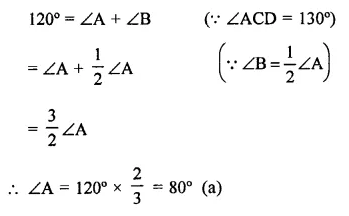Question 6 :
In ∆ABC, ∠B = ∠C and ray AX bisects the exterior angle ∠DAC. If ∠DAX = 70°, then ∠ACB =
(a) 35°
(b) 90°
(c) 70°
(d) 55°

In ∆ABC, ∠B = ∠C
AX is the bisector of ext. ∠CAD
∠DAX = 70°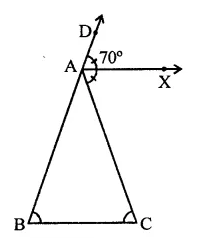∴ ∠DAC = 70° x 2 = 140°
But Ext. ∠DAC = ∠B + ∠C
= ∠C + ∠C (∵ ∠B = ∠C)
= 2∠C
∴ 2∠C = 140° ⇒ ∠C =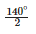= 70°
∴ ∠ACB = 70° (c)

Question 7 :
In a triangle, an exterior angle at a vertex is 95° and its one of the interior opposite angle is 55°, then the measure of the other interior angle is
(a) 55°
(b) 85°
(c) 40°
(d) 9.0°

In ∆ABC, BA is produced to D such that ∠CAD = 95°
and let ∠C = 55° and ∠B = x°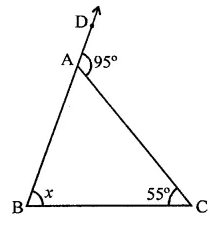∵ Exterior angle of a triangle is equal to the sum of its opposite interior angle
∴ ∠CAD = ∠B + ∠C ⇒ 95° = x + 55°
⇒ x = 95° – 55° = 40°
∴ Other interior angle = 40° (c)

Question 8 :
If the sides of a triangle are produced in order, then the sum of the three exterior angles so formed is
(a) 90°
(b) 180°
(c) 270°
(d) 360°

In ∆ABC, sides AB, BC and CA are produced in order, then exterior ∠FAB, ∠DBC and ∠ACE are formed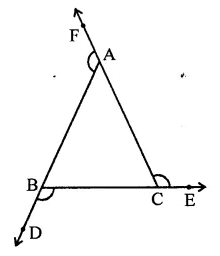We know an exterior angles of a triangle is equal to the sum of its interior opposite angles
∴ ∠FAB = ∠B + ∠C
∠DBC = ∠C + ∠A and
∠ACE = ∠A + ∠B Adding we get,
∠FAB + ∠DBC + ∠ACE = ∠B + ∠C + ∠C + ∠A + ∠A + ∠B
= 2(∠A + ∠B + ∠C)
= 2 x 180° (Sum of angles of a triangle)
= 360° (d)

Question 9 :
In ∆ABC, if ∠A = 100°, AD bisects ∠A and AD⊥ BC. Then, ∠B =
(a) 50°
(b) 90°
(c) 40°
(d) 100°

In ∆ABC, ∠A = 100°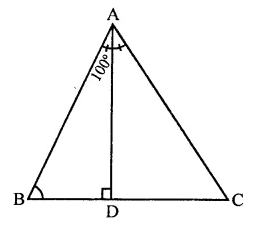Now, ∠BAD =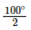= 50°
In ∆ABD,
∠BAD + ∠B + ∠D= 180°
(Sum of angles of a triangle)
⇒ ∠50° + ∠B + 90° = 180°
∠B + 140° = 180°
⇒ ∠B = 180° – 140° ∠B = 40° (c)

Question 10 :
An exterior angle of a triangle is 108° and its interior opposite angles are in the ratio 4:5. The angles of the triangle are
(a) 48°, 60°, 72°
(b) 50°, 60°, 70°
(c) 52°, 56°, 72°
(d) 42°, 60°, 76°

In ∆ABC, BC is produced to D and ∠ACD = 108°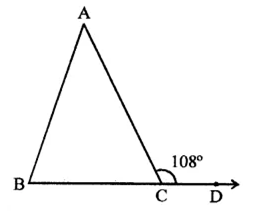Ratio in ∠A : ∠B = 4:5
∵ Exterior angle of a triangle is equal to the sum of its opposite interior angles
∴ ∠ACD = ∠A + ∠B = 108°
Ratio in ∠A : ∠B = 4:5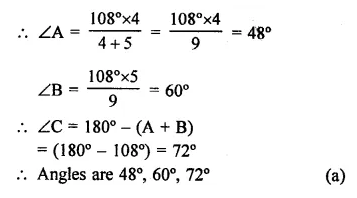Todays Deals### RD Chapter 11- Co-ordinate Geometry Ex-MCQS Contributorskrishan

Name:
Email:

# Latest News# 9000 interview questions in different categories